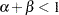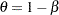BOUNDS Statement

BOUNDS inequality <, ..., inequality> ;

The BOUNDS statement restricts the parameter estimates so that they lie within specified regions. In each BOUNDS statement, you can specify a series of boundary values separated by commas. The series of bounds is applied simultaneously. Each boundary specification consists of a list of parameters, an inequality comparison operator, and a value. In a single-bounded expression, these three elements follow one another in the order described. The following are examples of valid single-bounded expressions:

bounds a1-a10 <= 20;
bounds c > 30;
bounds a b c > 0;

Multiple-bounded expressions are also permitted. For example:

bounds 0 <= B<= 10;
bounds 15 < x1 <= 30;
bounds r <= s <= p < q;

If you need to restrict an expression involving several parameters (for example,), you can reparameterize the model so that the expression becomes a parameter or so that the boundary constraint can be expressed as a simple relationship between two parameters. For example, the boundary constraintin the model

model y = alpha + beta*x;

can be achieved by parameterizingas follows:

bounds alpha < theta;
model y = alpha + (1-theta)*x;

Starting with SAS 7.01, Lagrange multipliers are reported for all bounds that are enforced (active) when the estimation terminates. In the "Parameter Estimates" table, the Lagrange multiplier estimates are identified with names Bound1, Bound2, and so forth. An active bound is treated as if a restriction were applied to the set of parameters so that one parameter degree of freedom is deducted. You can use the UNCORRECTEDDF option to prevent the loss of degrees of freedom when bounds are active.